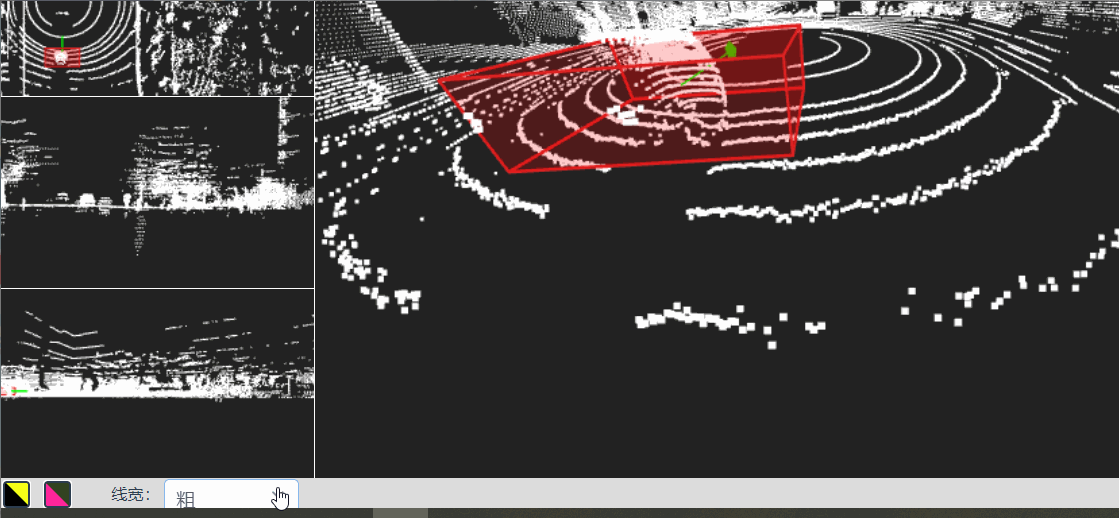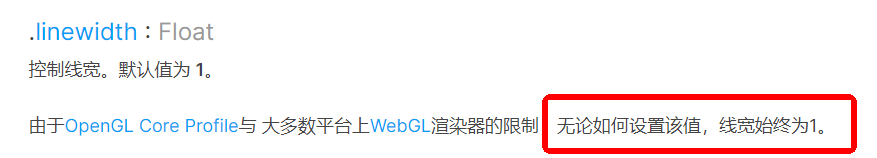# Three.js之绘制物体的边框及修改lineWidth``````// 引入新的线的材质类
import { LineMaterial } from 'three/examples/jsm/lines/LineMaterial.js';
import { LineGeometry } from 'three/examples/jsm/lines/LineGeometry.js';
import { Line2 } from 'three/examples/jsm/lines/Line2.js';

// 设置边框
const edgesGeometry = new LineGeometry();
const pointArr = this.getLinePoint(points);
const lineMaterial = new LineMaterial({
color: 0xdd2222,
linewidth: 2, // 这里表示线的宽度
transparent: true
});
edgesGeometry.setPositions(pointArr);
lineMaterial.resolution.set(window.innerWidth, window.innerHeight);
``````

``````// 获取边框的点连线数据
getLinePoint(points): Array<any> {
// 立方体的8个顶点坐标
let [p0, p1, p2, p3, p4, p5, p6, p7] = points;

//顺着点 保证12条线都连上即可
let pointLine = [
p0, p1, p2, p3, p0,
p4, p5, p6, p7, p4,
p4, p7, p3, p2, p6, p5, p1
];
let arr = [];
pointLine.forEach(item => {
arr.push(item.x)
arr.push(item.y)
arr.push(item.z)
})
return arr;
}
``````